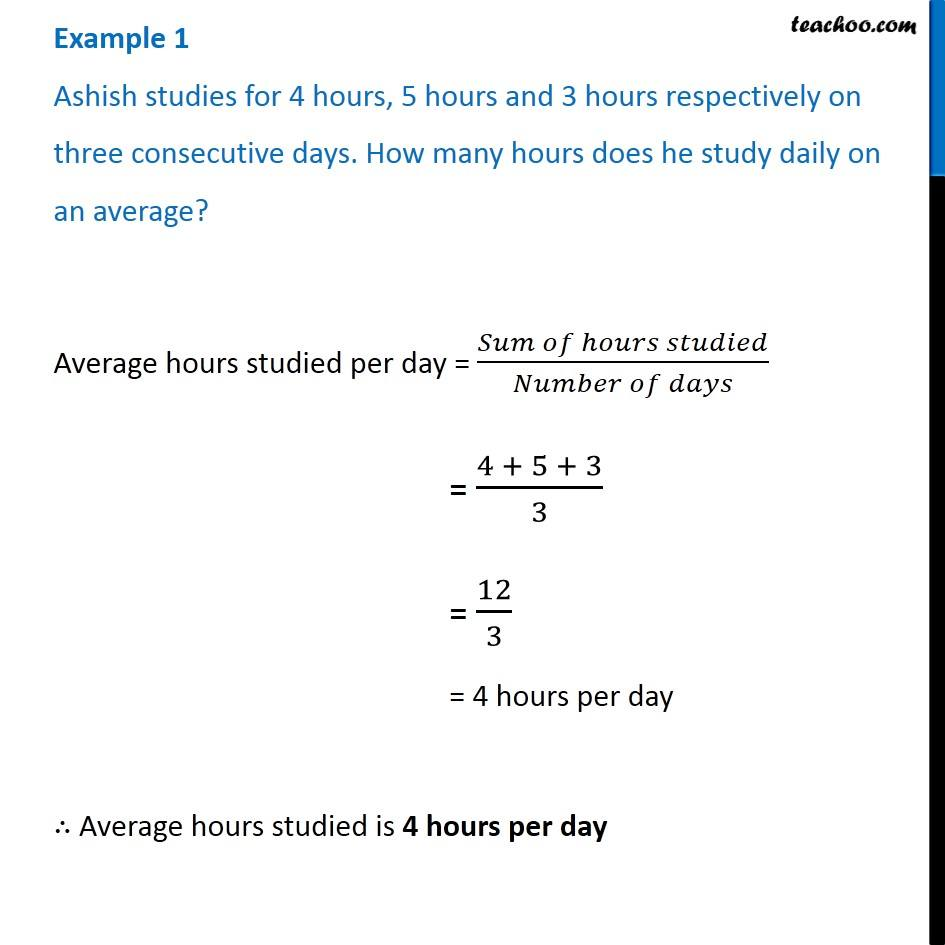Examples

Chapter 3 Class 7 Data Handling
Serial order wise### Transcript

Example 1 Ashish studies for 4 hours, 5 hours and 3 hours respectively on three consecutive days. How many hours does he study daily on an average? Average hours studied per day = (𝑆𝑢𝑚 𝑜𝑓 ℎ𝑜𝑢𝑟𝑠 𝑠𝑡𝑢𝑑𝑖𝑒𝑑)/(𝑁𝑢𝑚𝑏𝑒𝑟 𝑜𝑓 𝑑𝑎𝑦𝑠) = (4 + 5 + 3)/3 = 12/3 = 4 hours per day ∴ Average hours studied is 4 hours per day